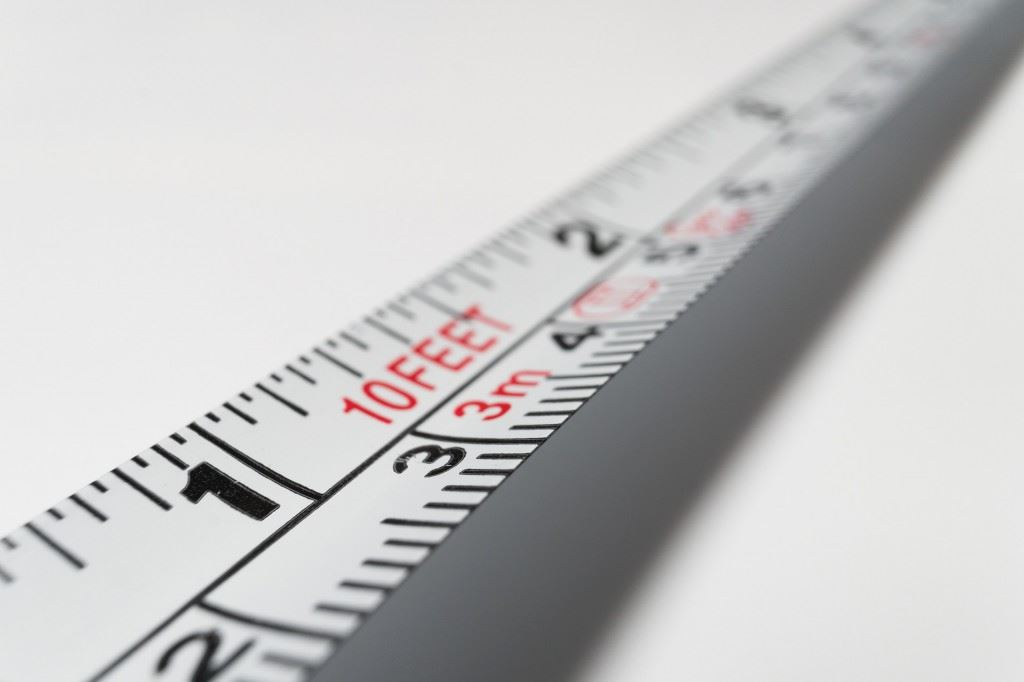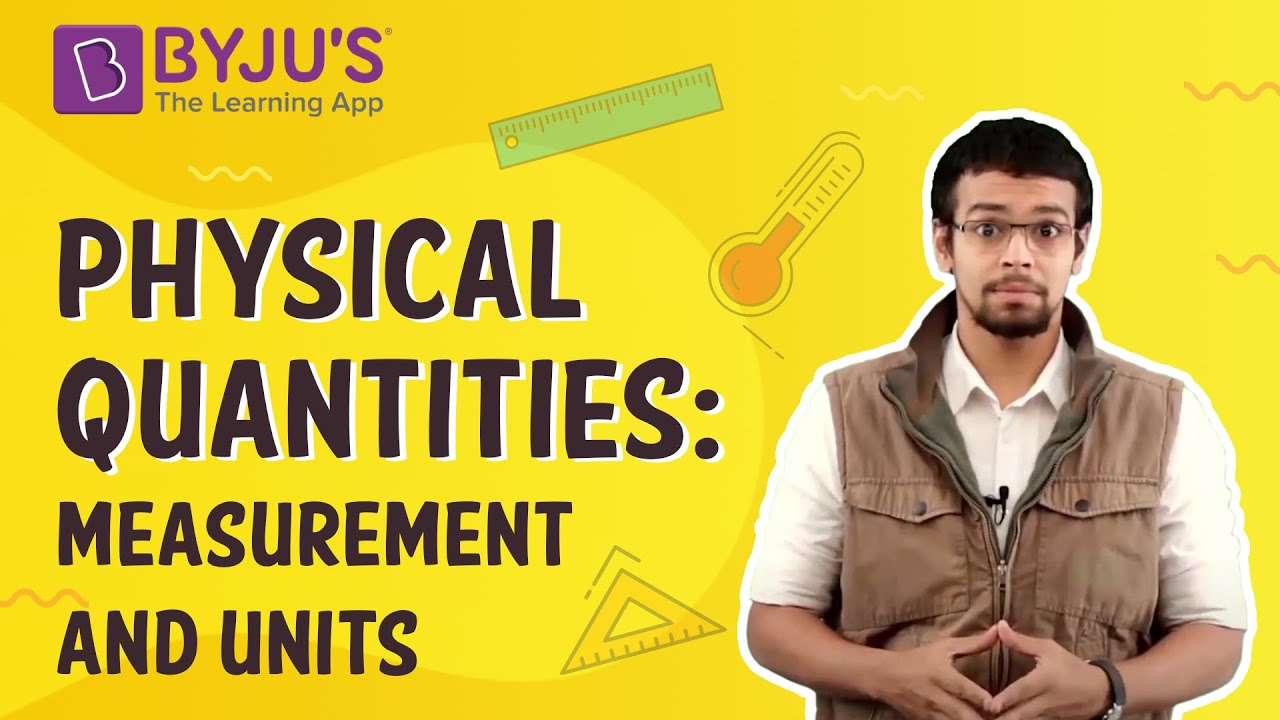# Errors In Measurement - Absolute Error and Relative Error

Any measurement that you make is just an approximation, 100% accuracy is not possible. If you measure the same object two different times, the two measurements may not be exactly the same.  The difference between the two measurements is called a variation in the measurements. This variation introduces an unwanted but unavoidable uncertainty in our measurement. This uncertainty is called the Errors in measurement. This ‘error’ should not be confused with a ‘mistake’. Error, unlike mistake, does not mean that you got the wrong answer. It just means you didn’t get as close to the true value as possible. The errors in measurement are a mathematical way to show the uncertainty in the measurement.  It is the difference between the result of the measurement and the true value of what you were measuring.Absolute and relative error are two types of error with which every experimental scientist should be familiar. The differences are important.

## How to find errors in measurement

### Tolerance/ Margin of Error

Error in measurement may be represented by a tolerance (margin of error).  Machines used in manufacturing often set tolerance intervals or ranges in which product measurements will be tolerated or accepted before they are considered flawed.

Tolerance refers to the greatest range of variation that can be allowed or is acceptable and it is one half of the precision of an instrument. So to obtain the range, we add and subtract one half of the precision of measuring instruments.

For example, if a ruler has to measure an object of which has a length of 5.4 cm and it measures this with a precision of 0.1 cm. This gives a tolerance interval in this measurement is 5.4 ± 0.05 cm, or from 5.35 cm to 5.45 cm.  Any measurements within this range are “tolerated” or perceived as correct.

## Absolute Error

Absolute error in measurement refers to the actual amount of error in the measurement. The absolute error of the measurement shows how large the error actually is. Absolute error is inadequate due to the fact that it does not give any details regarding the importance or the graveness of the error. If you are measure distances between cities kilometers apart, an error of a few centimeters is negligible and is irrelevant. On the other hand, an error of centimeters when measuring small machine parts is a very significant error. Both the errors are in the order of centimeters, but the second error is more severe than the first.

Absolute Error can be represented by the expression;

$$\begin{array}{l}E_{absolute}=|x_{measured}-x_ {accepted}|\end{array}$$

For example, if you know a length is 3.535 m + 0.004 m, then 0.004 m is an absolute error. The absolute error is the difference between the measured value and the actual value.

## Relative Error

Relative error represents the ratio of the absolute error of the measurement to the accepted measurement. This way we can determine the magnitude of the absolute error in terms of the actual size of the measurement. If the true measurement of the object is not known then the relative error is found using the measured value. The expression for relative error is;

$$\begin{array}{l}E_{relative}=\frac{E_{absolute}}{x_{accepted}}\end{array}$$

$$\begin{array}{l}Relative\; Error=\frac{|measured\; value-actual\; value|}{actual\; value}\end{array}$$

## Percentage Error

Errors in measurement may also be expressed in terms of percentage. This is similar to relative error except that the error here is converted to a percent value. The percentage error is found by multiplying the relative error by 100%.

$$\begin{array}{l}percent\; of\; Error=\frac{|measured\; value-actual\; value|}{actual\; value}*100\end{array}$$
%

This brings us to an end on the article on absolute error, relative error, and tolerance, the major ways of expressing errors in measurements. To learn more related topics like significant figures please visit BYJU’S.

## Watch the video to find out what are base measurements?Test Your Knowledge On Errors Absolute Error Relative Error!